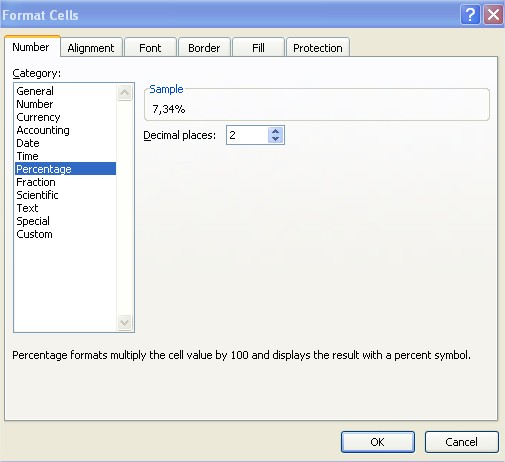### How to calculate ROI in Excel

In this lesson you can learn how to calculate ROI in Excel.

ROI stands for return on investment. Return of investment is one of the most basics measures in the business. ROI is a profitability indicator which is used to measure the efficiency of the company, regardless of the structure of its assets or extraordinary factors. Return of investment is also helpful for project managers because ROI helps project managers to calculate the efficiency of the project.

Let’s build the return on investment calculator in Excel. To calculate ROI in Excel first you need some data. You need net profit and cost of investment.

Copy and paste this roi formula in cell B4: =B2/B3This formula will calculate the ROI for the investment data you place in cells B2 and C2 and is based on roi equation formula:

ROI = Net Profit / Cost of Investment

Remember to format ROI as Percentage. Click B4 cell > click CTRL + 1 keyboard shortcut > click Percentage with 2 decimal places## Template

```Further reading: